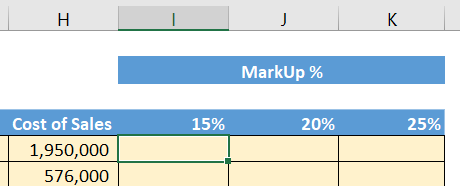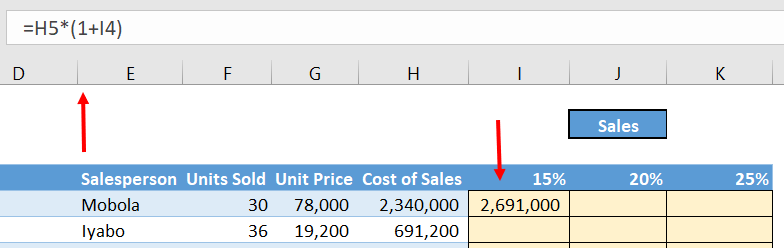•#### Hands-On Financial Modeling with Microsoft Excel 2019#### Overview of this book

Financial modeling is a core skill required by anyone who wants to build a career in finance. Hands-On Financial Modeling with Microsoft Excel 2019 explores terminologies of financial modeling with the help of Excel. This book will provides you with an overview of the steps you should follow to build an integrated financial model. You will explore the design principles, functions, and techniques of building models in a practical manner. Starting with the key concepts of Excel, such as formulas and functions, you will learn about referencing frameworks and other advanced components for building financial models. Later chapters will help you understand your financial projects, build assumptions, and analyze historical data to develop data-driven models and functional growth drivers. The book takes an intuitive approach to model testing and covers best practices and practical use cases. By the end of this book, you will have examined the data from various use cases, and have the skills you need to build financial models to extract the information required to make informed business decisions.
PrefaceFree Chapter
Section 1: Financial Modeling - OverviewIntroduction to Financial Modeling and ExcelSteps for Building a Financial ModelSection 2: The Use of Excel - Features and Functions for Financial ModelingFormulas and Functions - Completing Modeling Tasks with a Single FormulaApplying the Referencing Framework in ExcelSection 3: Building an Integrated Financial ModelUnderstanding Project and Building AssumptionsAsset and Debt SchedulesCash Flow StatementValuationRatio AnalysisModel Testing for Reasonableness and AccuracyAnother Book You May Enjoy# Mixed referencing

The following example, which is using the same Sales Report worksheet, seeks to compare sales that are obtained by using MarkUps of 15%, 20%, and 25%.

In this case, the sales is calculated as Cost of Sales × (1+MarkUp %). This is a screenshot of the layout for the markup:Mixed referencing is required when you need to lock a reference in one direction only, either down or across to the right, but not both. In the following example, you will create the formula in cell I5, and then copy it down through rows 6 to 20 and across columns J and K. The following screenshot shows the calculation of 15% MarkUp:The base formula is H5*(1+I4).

Note that there are two cell references in the formula, H5 and I4, which you will need to consider individually.

Cell H5 is the Cost of Sales. The column part is H, which we will look at when we consider copying to the right...